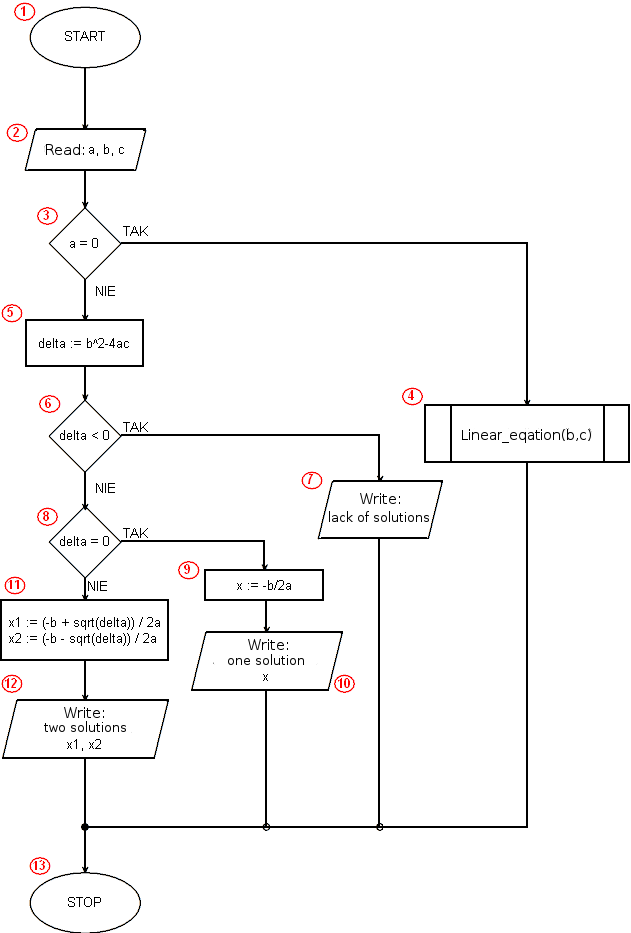# Implementation of the algorithm solving a quadratic equation in Pascalprogram Quadratic_equation;

procedure linear(a: double; b: double);
var x: double;
begin
if a=0 then
if b=0 then
writeln('identity equation')
else
writeln('contrary equation')
else begin
x:=-b/a;
writeln('Linear equation, x = ',x);
end;
end;

var a,b,c,delta,x,x1,x2: double;
begin
write('a = ');
write('b = ');
write('c = ');
if a=0 then
linear(b,c)
else begin
delta:=b*b-4*a*c;
if delta<0 then
writeln('lack of solutions')
else
if delta=0 then begin
x:=-b/(2*a);
writeln('one solution x = ',x);
end else begin
x1:=(-b+sqrt(delta))/(2*a);
x2:=(-b-sqrt(delta))/(2*a);
writeln('x1 = ',x1);
writeln('x2 = ',x2);
end;
end;
end.

 Program line Description Block algorithm 1 Every program in the pascal must start with the keyword program preceding the name of the program, it adds nothing to the implementation of the algorithm. - 3 - 15 We declare and define a linear procedure that implements the algorithm of solving a linear equation. 4 17 We declare all variables used in the main program. In a pascal, we must do this before the keyword begin, which starts the operating part of the code. - 18 The beginning of the program code. 1 19 - 24 On the screen, we print the prompts for the user and load the values of the coefficients a, b and c entered into the corresponding, previously declared, variables. 2 25 We check if the coefficient a is equal to 0. If this is the case with the linear equation. 3 26 In this line we will only find ourselves if a = 0, ie when the equation is a linear equation. We invoke procedure linear(b, c) that realizes the algorithm of solving a linear equation. It is worth noting that the coefficient at the first power of the variable x is marked with the symbol b, and the free expression c (traditionally in the linear equation, a and b are used respectively). 4 27 else, i.e. otherwise. At this point, the solution of the equation begins, when a ≠ 0, in other words when we actually deal with a quadratic equation. - 28 We calculate the value of the delta variable. 5 29 We check if delta < 0. 6 30 When delta < 0, we print an appropriate message about the lack of solutions. 7 31 Here the program block begins when the delta is not less than zero, ie when the equation has solutions (one or two). - 32 We check if delta = 0. 8 33 We calculate and store in the x variable the solution of the equation (single, double - delta=0). 9 34 We display the calculated result. 10 35 We start the program block executed in the situation when the condition delta = 0 (and earlier delta <0 and a ≠ 0) was not met. - 36 - 37 We calculate solutions and their values we assign to variables x1 and x2. 11 38 - 39 We display the calculated result - two solutions of the quadratic equation. 12 40 - 41 We close the blocks of compound instructions. - 42 The end of the program implementation. 13